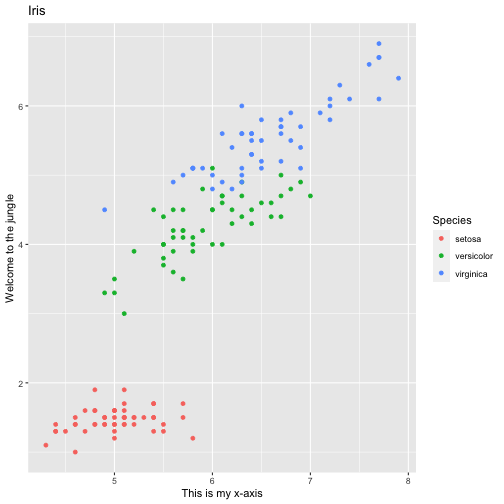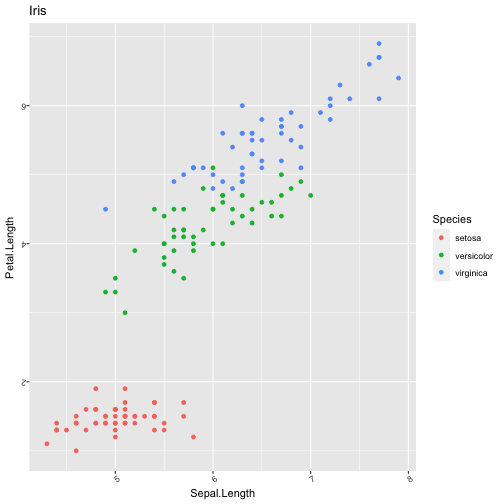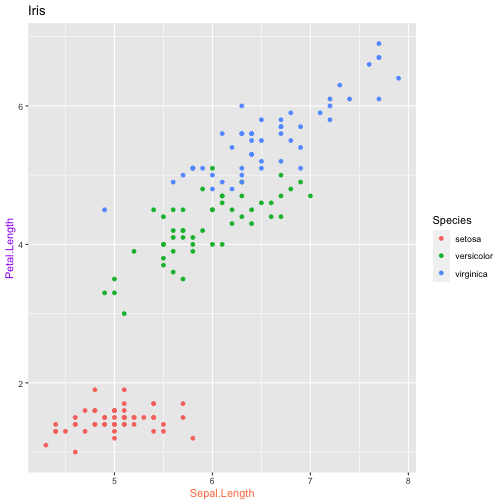Text

There are various ways to set the formatting of labels in plots. First and foremost, you may want to change the label text:

ggplot(data=iris,
mapping=aes(x=Sepal.Length,
y=Petal.Length, color=Species))+
ggtitle("Iris")+
geom_point()+
gg_("set the x-axis label to 'This is my x-axis'")+
gg_("The y-axis label should be set to 'Welcome to the jungle', please")Rotation

ggplot(data=iris,
mapping=aes(x=Sepal.Length,
y=Petal.Length, color=Species))+
ggtitle("Iris")+
geom_point()+
gg_("Rotate the x-axis labels by 32°")+
gg_("Rotate the y-axis labels by 172 degrees")Colors

ggplot(data=iris,
mapping=aes(x=Sepal.Length,
y=Petal.Length, color=Species))+
ggtitle("Iris")+
geom_point()+
gg_("Paint the x-axis label in coral.")+
gg_("The label of the y-axis should appear in purple.")